#Interactive Real Analysis

## 8. Sequences of Functions

### 8.2. Uniform Convergence

We saw in the previous section that pointwise convergence of a sequence of functions was easy to define, but was too simplistic of a concept. We would prefer a type of convergence that preserves at least some of the shared properties of a function sequence. Such a concept is uniform convergence.

 Definition 8.2.1: Uniform Convergence A sequence of functions { fn(x) } with domain D converges uniformly to a function f(x) if given any> 0 there is a positive integer N such that | fn(x) - f(x) | <for all xD whenever nN Please note that the above inequality must hold for all x in the domain, and that the integer N depends only on.

We should compare uniform with pointwise convergence:

• For pointwise convergence we could first fix a value for x and then choose N. Consequently, N depends on bothand x.
• For uniform convergence fn(x) must be uniformly close to f(x) for all x in the domain. Thus N only depends onbut not on x.

Let's illustrate the difference between pointwise and uniform convergence graphically:

Pointwise Convergence Uniform Convergence

For pointwise convergence we first fix a value x0. Then we choose an arbitrary neighborhood around f(x0), which corresponds to a vertical interval centered at f(x0).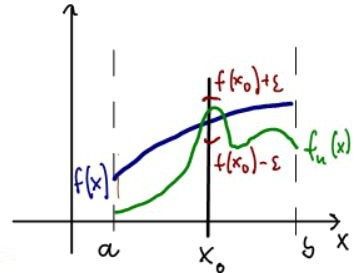Finally we pick N so that fn(x0) intersects the vertical line x = x0 inside the interval (f(x0) -, f(x0) +)

For uniform convergence we draw an-neighborhood around the entire limit function f, which results in an "-strip" with f(x) in the middle.Now we pick N so that fn(x) is completely inside that strip for all x in the domain.

 Example 8.2.2: Pointwise versus Uniform ConvergenceDefine fn(x) = x/n with domain D = [a, b]. Show that the sequence { fn(x) } converges uniformly to zero. What if we change the domain to all real numbers?Let fn(x) = xn with domain D = [0, 1]. Show that { fn(x) } converges pointwise but not uniformly. What if we change the domain slightly to D = (0, 1)?The sequence fn(x) = max(n - n2 |x - 1/n|, 0) with domain D = [0, 1] converges pointwise to zero. Does it also converges uniformly?Remember that we discussed uniform continuity in a previous chapter. We showed that a function that is (regularly) continuous on a compact set is automatically uniformly continuous. Is that true also for pointwise and uniform convergence, i.e. is a sequence that converges pointwise on a compact set automatically uniformly convergent?Can you find a uniformly convergent sequence for all real numbers?

Uniform convergence clearly implies pointwise convergence, but the converse is false as the above examples illustrate. Therefore uniform convergence is a more "difficult" concept. The good news is that uniform convergence preserves at least some properties of a sequence.

 Theorem 8.2.3: Uniform Convergence preserves Continuity If a sequence of functions fn(x) defined on D converges uniformly to a function f(x), and if each fn(x) is continuous on D, then the limit function f(x) is also continuous on D.

Before we continue, we will introduce a new concept that will somewhat simplify our discussion of uniform convergence, at least in terms of notation: we will use the supremum of a function to define a 'norm' of f.

 Definition 8.2.4: Supremum Norm Suppose f: D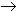R is a function with domain D. We define the sup-norm of f over D as: || f ||D = sup{ |f(x)|: xD } If the context is clear, we simply write || f ||.

Clearly, a function f is bounded if and only if || f || <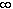, which follows immediately from the various definitions. The connection between sup-norm and uniform convergence is also straight-forward.

 Proposition 8.2.5: Sup-norm and Uniform Convergence A sequence fn: DR converges uniformly to f: DR if and only if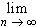||fn - f||D = 0

Using this proposition it is can be easy to show uniform convergence of a function sequence, especially if the sequence is bounded. Still, even with this idea of sup-norm uniform convergence can not improve its properties: it preserves continuity but has a hard time with differentiability.

 Example 8.2.6: Uniform Convergence does not imply DifferentiabilityShow that the sequence fn(x) = 1/n sin(n x) converges uniformly to a differentiable limit function for all x.For the above example, show that the sequence of derivatives fn' does not converge to the derivative of the limit function.Find a sequence of differentiable functions that converges uniformly to a continuous limit function but the limit function is not differentiable.

So uniform continuity is good enough to preserve continuity, but does not preserve differentiability. Our next question will of course be: what is the impact on integration? We already know that pointwise convergence does not preserve Riemann-integrability, and even if it does we can not switch limits and integration. What about uniform convergence?

 Theorem 8.2.7: Uniform Convergence and Integration Let fn(x) be a sequence of continuous functions defined on the interval [a, b] and assume that fn converges uniformly to a function f. Then f is Riemann-integrable andfn(x) dx =fn(x) dx =f(x) dx

Note: Much more can be said about convergence and integration if we consider the Lebesgue integral instead of the Riemann integral. To focus on Lebesgue integration, for example, we would first define the concept of "convergence almost everywhere":

 Definition 8.2.8: Convergence Almost Everywhere A sequence fn defined on a set D converges (pointwise or uniformly) almost everywhere if there is a set S with Lebesque measure zero such that fn converges (pointwise or uniformly) on D \ S. We say that fn converges (pointwise or uniformly) to f a.e.

In other words, convergence a.e. means that a sequence converges everywhere except on a set with measure zero. Since the Lebesgue integral ignores sets of measure zero, convergence a.e. is ready-made for that type of integration.

 Example 8.2.9: Convergence Almost Everywhere Let rn be the (countable) set of rational numbers inside the interval [0, 1], ordered in some way, and define the functions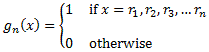and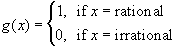Show the following: The sequence gn converges pointwise to g but the sequence of Riemann integrals of gn does not converge to the Riemann integral of g. The sequence gn converges a.e. to zero and so does the sequence of Lebesgue integrals of gn.

There are many theorems relating convergence almost everywhere to the theory of Lebesgue integration. They are too involved to prove at our level but they would certainly be on the agenda in a graduate course on Real Analysis. For us we will be content stating, without proof, one of the major theorems: the Lebesgue bounded convergence theorem.

 Theorem 8.2.10: Lebesgue's Bounded Convergence Theorem Let { fn } be a sequence of (Lebesgue) integrable functions that converges almost everywhere to a measurable function f. If |fn(x)|g(x) almost everywhere and g is (Lebesgue) integrable, then f is also (Lebesgue) integrable and: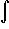| fn - f | dm = 0

It remains to clarify the connection between uniform convergence and differentiability. Simple - or pointwise - convergence is not enough to preserve differentiability, and neither is uniform convergence by itself. However, if we combine pointwise with uniform convergence we can indeed preserve differentiability and also switch the limit process with the process of differentiation.

 Theorem 8.2.11: Uniform Continuity and Differentiation Let fn(x) be continuously differentiable functions defined on the interval [a, b]. If: the sequence fn converges pointwise to a function f, and the sequence of derivatives fn' converges uniformly Then f is differentiable and f'(x) =fn'(x) for all x[a, b]. In other words, the limit process and the differentiation process can be switched in this case.

One of the main applications of our theory of uniform convergence is going to be series of functions and in particular power series ... which we will cover next.